.css-4zleql{display:block;}My blogCracking the Nimbus Cipher with Differential Cryptanalysis

(PicoCTF ’21 — Clouds)

Dhrumil Patel
·Apr 26, 2021·

When did Goku create a cipher with his Nimbus Cloud??!In “Clouds” (PicoCTF ‘21), you’re provided with an implementation of a cipher and an interesting hint: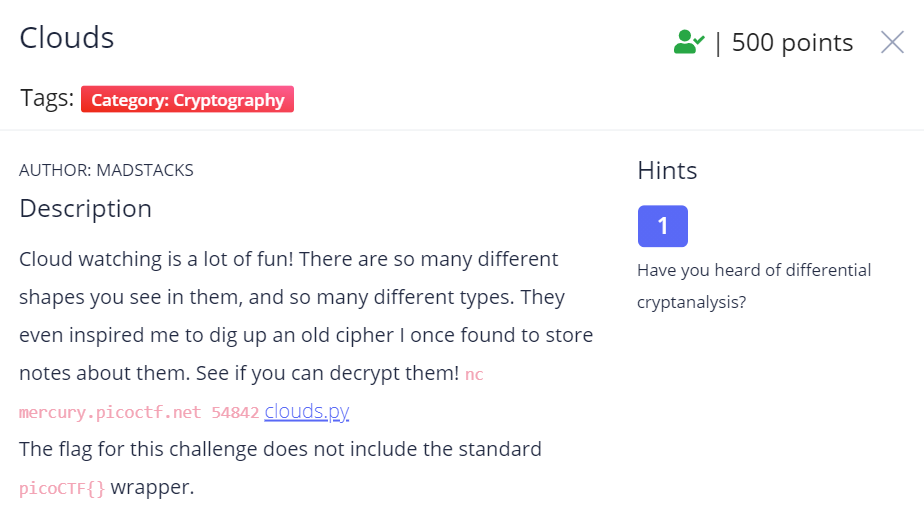Initial Impressions

When you run clouds.py, you see beautiful cloud art and a simple menu for storing and retrieving notes.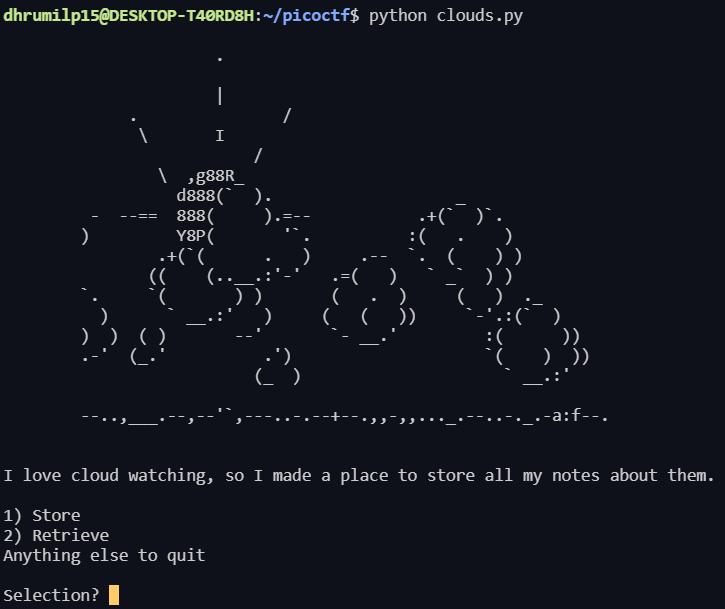After encrypting some plaintexts (we’ll call any input to the cipher a plaintext), we make a few observations:

• The cipher only accepts hex letters.
• The ciphertext (we’ll call any output from the cipher a ciphertext) is roughly the same length as the corresponding plaintext (16 letters).
• The ciphertext is also in hex letters.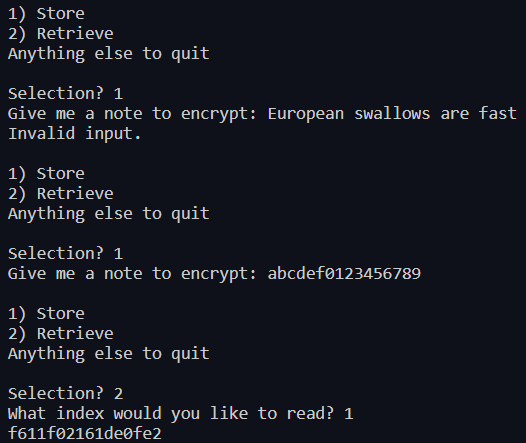Taking A Closer Look at the Cipher

The cipher maintains an array of ciphertexts — when we store a note, it’s encrypted using an encrypt function and is added to the end of the array.

In the encrypt function, plaintexts are encrypted in fixed-size blocks, which is a hallmark of a block cipher. Block ciphers encrypt fixed-size “blocks” (block sizes vary but they’re typically a power of 2 like 8 or 16) by iterating across the data. Data that doesn’t fill the block size is typically padded with null bytes (or zeros).

We notice the g function, which reverses the bits in a 64-bit number (i.e. a binary number ...110turns into 011...) and fills the right side with enough 0s to make the number 64 bits long. This g function provides the cipher with non-linearity, which makes it harder to crack.

There’s a round function, which is an operation that’s performed on plaintexts for several rounds to encrypt it. In the round function, a subkey (the key used in a particular round) is “mixed” into the plaintext —the subkey is added, multiplied and/or XORed with the plaintext. The real encryption in ciphers comes from keys “mixing” with the plaintext. Without key mixing, anyone can reverse a ciphertext and steal the plaintext.

The round function is technically the part inside the for loop In the round function, we see that the round’s subkey is XORed with result and the output of that operation is multiplied with an odd-ed version of the subkey. The cipher repeats this process for 5 rounds to encrypt a single block of the plaintext. After encrypting a block, the cipher moves on to the next block of data (or stops encrypting if the cipher reaches the end of the data). We can summarize the round function as this equation:Finally! The Attack!

After researching differential cryptanalysis, we discover that this cipher is the Nimbus Cipher, a block cipher that Alex Machado proposed to the NESSIE project.

It wasn’t accepted, but that might be because Vladimir Furman broke it with the attack he describes in Differential Cryptanalysis of Nimbus.

In his paper, Furman shows that the cipher’s subkeys can be recovered with 256 plaintexts and by testing 2¹⁰ subkeys. We’ll be using a variant on his attack that simplifies the approach in his paper (Rest assured, this approach still recovers the subkeys).

Most attacks that use differential cryptanalysis are chosen plaintext attacks. That means that we’ll design special plaintexts, encrypt them and analyze the resulting ciphertexts to recover information about the cipher. For our attack, we’ll design special pairs of plaintexts and analyze the resulting pairs of ciphertexts to recover all 5 subkeys.

Our attack will follow these steps:

1. Find a Differential Characteristic
2. Trace the characteristic through a smaller part of the cipher
3. Build the full path and recover each round’s subkey

Step 1: What’s a Differential Characteristic??

A differential characteristic is a property of the cipher that we exploit to determine the “state” of our plaintexts.

Suppose you encrypt 100 pairs of numeric plaintexts that have an XOR difference of 5 (i.e. the first number XORed with the second is equal to 5). If you can determine — with greater than random probability — how a pair of plaintexts is transformed within the cipher (e.g. your pairs always have an XOR difference of 7 after the g function), you’ve determined a state of the cipher (e.g. the state after the g function) and have found a differential characteristic.

For the Nimbus Cipher, we can rely on Furman’s work for the input differential (We’ll call this the “differential” and it’s the XOR difference of the plaintexts). Furman uses 2⁶³-2 as his input differential. Why? Let’s refer to Multiplicative Differentials by UC Berkeley researchers Nikita Borisov, Monica Chew, Rob Johnson, and David Wagner for an explanation.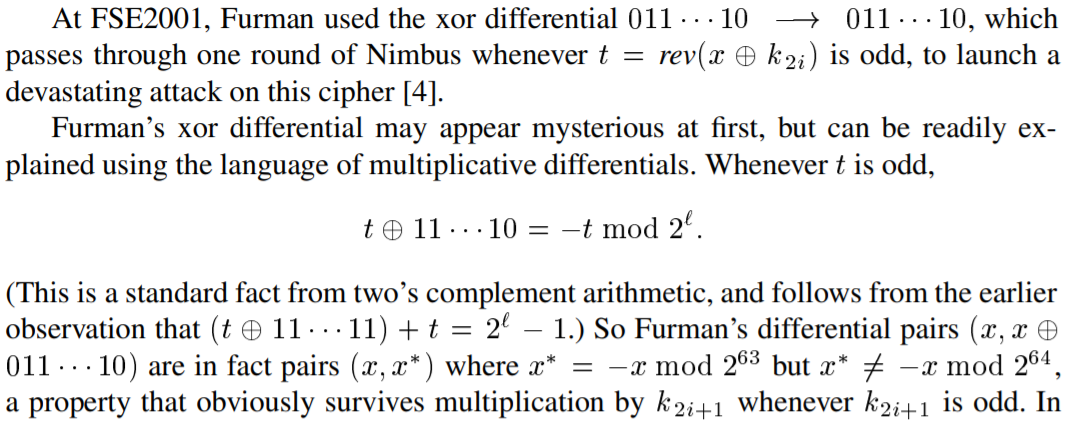Their explanation effectively states that odd outputs of the g function (t in the paper) are equal to their negative (modulo 2⁶⁴) when XORed with the differential. They then show that the pairs of plaintexts used in the attack have the same property (without the XOR) (modulo 2⁶³). The different bases (2⁶⁴ vs. 2⁶³ in the modulo equations) become more important later on since we can’t directly determine the value of a subkey’s most significant bit (the bit with the highest place value).

This property survives multiplication by the odd-ed subkey because it’s equivalent to (t ^ 11...11) + t= 2^64 — 1 (The ^ symbol means xor). Their paper actually goes further with this idea with multiplicative differentials, but we’ll leave that for a future post!

🎉🎉We have our differential characteristic, which means that we’ve completed the first step of our differential cryptanalysis attack. 🎉🎉

With the differential characteristic, let’s design our 256 plaintexts. We’ll generate 128 pairs of plaintexts that each have an XOR difference of the differential. Some pairs have certain characteristics according to Furman’s analysis.

Step 2: Trace the differential through a smaller part of the cipher

Furman also shows that the odd-ed subkey of the 5th round satisfies the equation below.In his paper, he uses this equation with ciphertexts that have an XOR difference of our differential on the 4th round but not the 5th round. We’ll calculate subkeys using the pair of ciphertexts and have each of the 128 pairs of ciphertexts “vote” on the correct subkey. We could choose to only use ciphertexts that satisfy the condition in his paper, but we’re able to circumvent that requirement.

This isn’t explicitly mentioned in the paper, but the multiplication between the subkey and the differential is modulo ²⁶⁴ because Nimbus operates on 64-bit numbers. I didn’t catch that at first, but I luckily discussed this problem with “robotabc773”, whose insight was invaluable to understanding this step.

When I first saw this equation, I was excited! I thought it would be trivial to isolate the subkey — I mean, one equation; one variable, how hard could it be?!

It turned out to be very hard😞. My first thought was to multiply both sides by the “modular multiplicative inverse” of the differential (imagine “division” but for modular multiplication), but that fails because a unique modular multiplicative inverse of our differential does not exist😞.

In fact, a unique modular multiplicative inverse of an integer and a base only exists if the integer and the base are coprime (the two integers share no common factors), but our base (2⁶⁴) and our differential (2⁶³ — 2), share 2 as a common factor. (Thanks to AdnanSlef for noticing that I accidentally wrote 2⁶³ — 1 here instead of 2⁶³ — 2).

With more research, we discover another way to solve linear congruences, which is just another name for these kinds of equations.

The key observation is that the solution for any linear congruence ax = b (mod m) is the same as the solution for ax — my = b, which we can solve using the extended euclidean algorithm. The solution to this equation is the modular multiplicative inverse of the differential (mod 2⁶³) rather than mod 2⁶⁴. With this solution, we won’t know the MSB of the subkey, but we’ll verify the subkey’s MSB later on by comparing decrypted ciphertexts and their corresponding plaintexts.

We find the modular multiplicative inverse of the differential (mod 2⁶³) and use it to solve for the subkey:

We return the most often calculated subkey from the ciphertexts. If all of the calculated subkeys seem equally likely (every ciphertext “voted” for a different subkey) or there are no subkeys, we know that the current arrangement of subkeys is invalid and we can move on to test another arrangement of subkeys.

Since we don’t know the MSB of the calculated subkey, we try both a 0 and a 1 for the MSB and add it to our subkey candidates. We also don’t know if the subkeys are actually even, so we’ll consider even versions of the subkey candidates.

Step 3: Recovering All 5 Subkeys!

With our candidates for the subkey of the 5th round, we can partially decrypt the ciphertexts and move on to the 4th round. Furman’s paper explains an algorithm that finds both the previous round’s odd-ed subkey as well as the current round’s subkey, but we’ll skip recalculating the 5th round’s odd-ed subkey and use the one we just calculated.

After decrypting the ciphertexts, we observe that we can apply the equation used to find the 5th round’s odd-ed subkey to find the 4th round’s odd-ed subkey.

Better yet, we can recover each round’s subkey with that equation!

We perform a recursive search of all of the possible keys (depth-first search). We’ll search through 4⁵ or 1024 subkeys and verify the correct arrangement of subkeys by comparing the decrypted ciphertexts and plaintexts. 🎉🎉Celebrate, because we’ve reached the end!🎉🎉

Step 4: The Hidden Step — Profit!!

With the subkeys in hand, all that’s left is to decrypt the flag!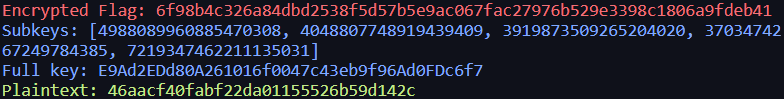After submitting the flag to the PicoCTF platform, you should welcome the overwhelming relief, because we’ve conquered the supposedly hardest cryptography challenge of PicoCTF ‘21.

Thanks for reading! If you liked my write-up and explanation, I’d appreciate claps/reactions! If you didn’t like it, feel free to comment below and we can improve it together! This challenge consumed my mind for 2 weeks, even after the contest officially ended — it was a weird moment when the cipher entered my dreams… I’ve included the full attack below.

The Full Attack

The first parts of the code are primarily from the provided implementation (excuse the import statements) but the rest is the attack.

Feel free to post your questions below or email me at ! (If you just want to talk, email me or message me on discord at dhrumilp15#4369) If you’d like to see my other math-y posts, check out my profile, website, GitHub or my last article about a Jane Street Puzzle!